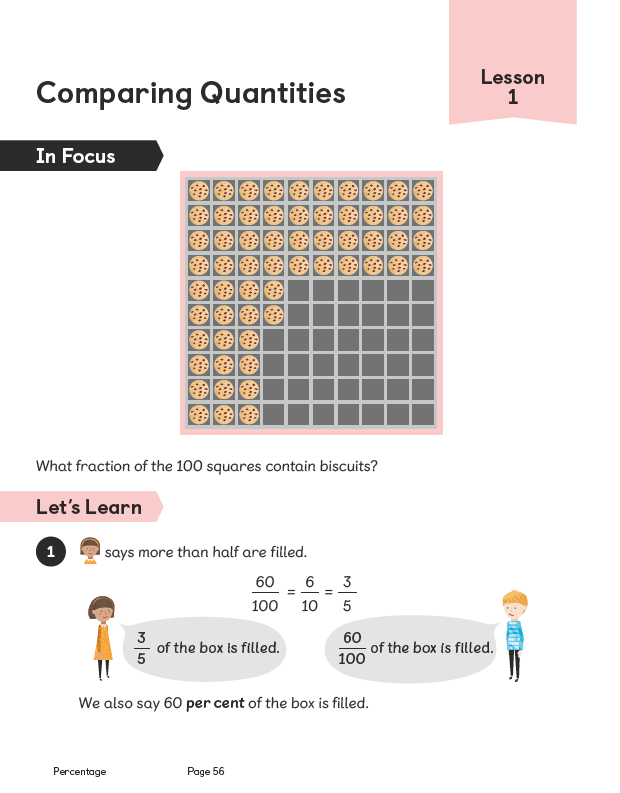Maths

What will we be learning in mathematics in Y5 this year-Fractions and Decimals

We are already over halfway through our Fractions chapter 6 topic which has covered equivalent fractions, converting mixed numbers to improper fractions and vice versa and adding and subtracting fractions. We will also be covering multiplying and dividing fractions before half term break. After half term, we will be covering decimals - looking at place value, finding the fraction equivalent, ordering and rounding decimals and adding and subtracting decimal amounts. This will then be followed by Percentages - finding decimal and fraction equivalents and percentage amounts.

Phew! Spring maths is busy!Top